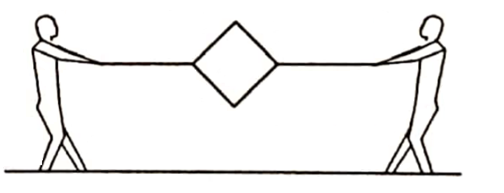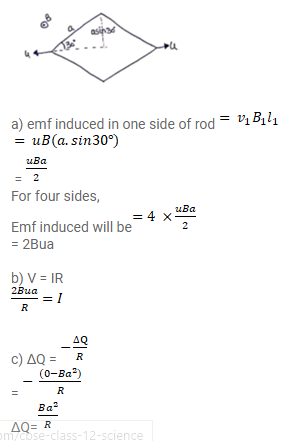# Figure shows a metallic square frame of edge a in a vertical plane.

Question:

Figure shows a metallic square frame of edge a in a vertical plane. A uniform magnetic field B exists in the space in a direction perpendicular to the plane of the figure. Two boys pull the opposite corners of the square to deform it into a rhombus. They start pulling the corners at $\mathrm{t}=0$ and displace the corners at a uniform speed $u$.

(a) Find the induced emf in the frame at the instant when the angles at these corners reduce to $60^{\circ}$.

(b) Find the induced current in the frame at this instant if the total resistance of the frame is $\mathrm{R}$.

(c) Find the total charge which flows through a side of the frame by the time the square is deformed into a straight line.Solution: